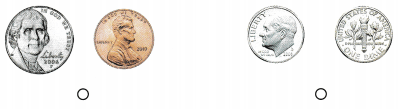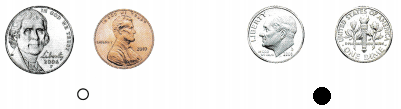# Texas Go Math Kindergarten Lesson 15.3 Answer Key Dime

Refer to our Texas Go Math Kindergarten Answer Key Pdf to score good marks in the exams. Test yourself by practicing the problems from Texas Go Math Kindergarten Lesson 15.3 Answer Key Dime.

## Texas Go Math Kindergarten Lesson 15.3 Answer Key Dime

Essential Question
How do you identify, describe, and name dimes?

Explore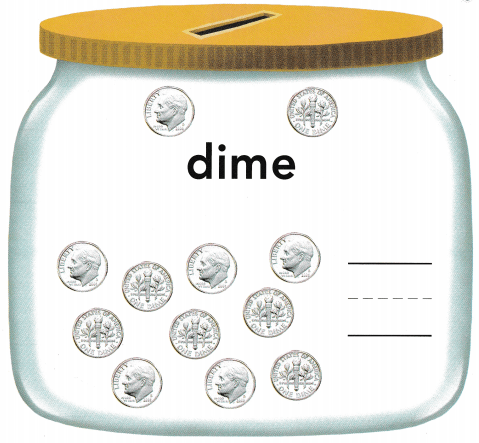Explanation:
Dime is a ten-cent coin
The person on the coin is Franklin D. Roosevelt, our 32nd president
He’s been on the dime since 1946.

Directions
Place a dime to match each one shown at the top of the page. Tell what is alike about the dimes. Tell what is different about the dimes. Count the set of dimes at the bottom of the page. Write the number of dimes.

Share and Show

Question 1.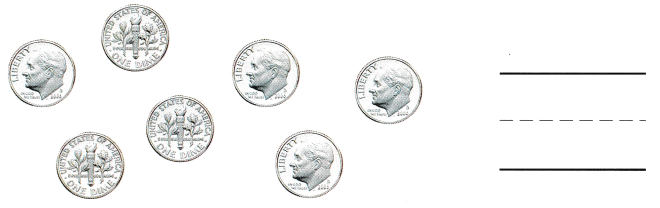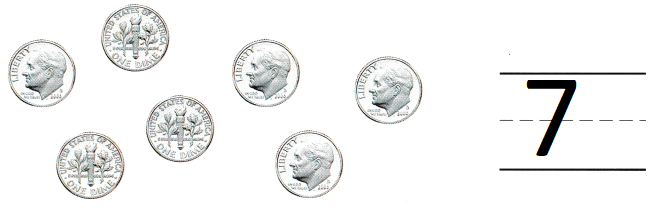Explanation:
A dime is worth 10 cents
There are 7 dimes

Question 2.Explanation:
A dime is worth 10 cents
there are 4 dimes

Question 3.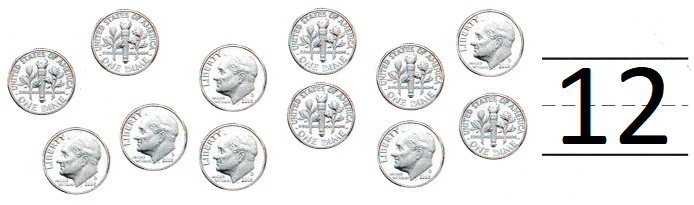Explanation:
A dime is worth 10 cents
There are 12 dimes.

Directions
1-3. Count the dimes. Write the number that shows how many dimes.

Question 4.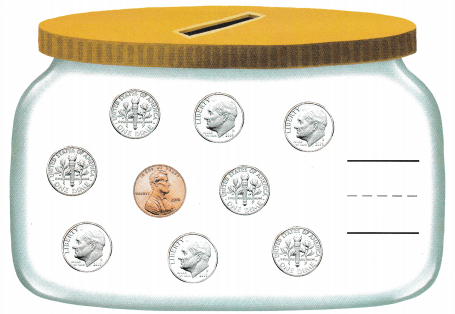Explanation:
A dime is worth 10 cents
There are 8 dimes

Question 5.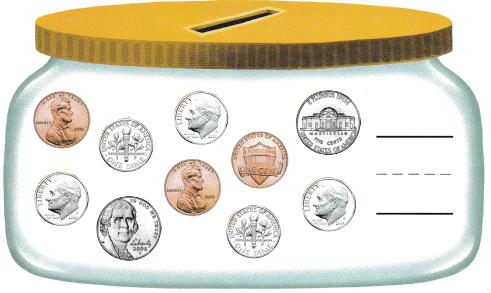Explanation:
A dime is worth 10 cents
There are 6 dimes

Directions
4-5. Circle the dimes. Count and write the number of dimes.

Home Activity

• Show your child a dime. Have him or her tell you the name of the coin and describe both sides.

Problem Solving

Question 6.Explanation:
Emmett needs 8 dimes to buy a toy.
But he has only 6 dimes
so, he doesn’t have enough money

Question 7.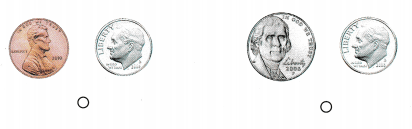Explanation:
Dana has a nickel and a dime.
so, marked the nickel and dime.

Directions
6. Emmett needs 8 dimes to buy a toy. Count the dimes to see if he has enough. Write the number of dimes Emmett has. Circle the dimes if he has enough money to buy a toy. 7. Choose the correct answer. Dana has a nickel and a dime. Which coins belong to Dana?

### Texas Go Math Kindergarten Lesson 15.3 Homework and Practice

Question 1.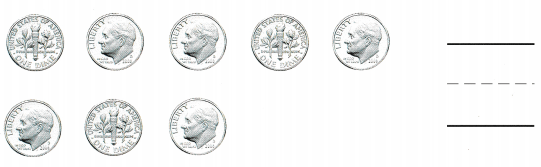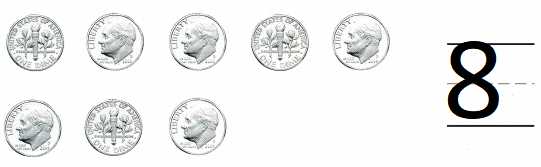Explanation:
A dime is worth 10 cents
There are 8 dimes

Question 2.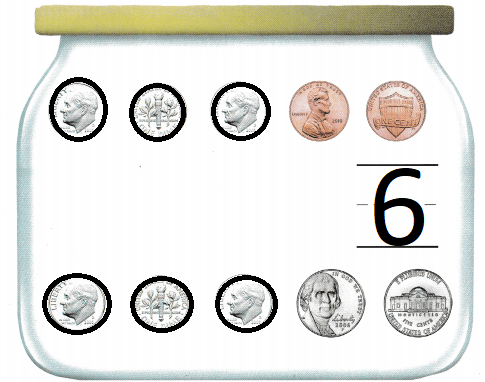Explanation:
A dime is worth 10 cents
There are 6 dimes

Directions
1. Count the dimes. Write the number that shows how many dimes. 2. Circle the dimes. Count and write the number of dimes.

Lesson Check

Question 3.Explanation:
Chang has a penny and a nickel,
Marked the coins that belongs to Chang

Question 4.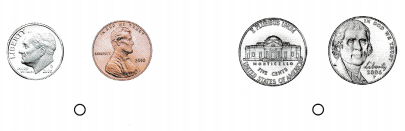Explanation:
Wendy has two nickels.
marked coins that belong to Wendy

Question 5.